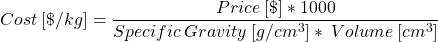# Calculator: Finding the ‘reel’ cost of volumetric filament pricing

Feb 18th, 2022

The cost of materials are an important factor when calculating a 3D printer’s total cost of ownership. Closed material systems, especially for industrial 3D printers, often mark up the cost of their materials, sometimes as much as 500% for simple commodity polymers. To help disguise the costs, these proprietary materials are often sold in volumetric (cubic centimeter / inch) increments instead of the industry standard weight-based pricing. So how do you convert this into a cost you can compare?

### How to Convert Cubic Centimeter Filaments to Kilograms for Cost Comparison

First, you will need to find the filament’s Material Data Sheet (MDS) or Technical Data Sheet (TDS). Here you will find the material’s density or specific gravity, often stated in grams per cubic centimeter. To help, we have provided a list of common materials and densities below for reference. Now that you have the density use the following equation or use the calculator below.### Calculate Cost

Convert cubic cm filament to cost per kg.

g/cm3
cm3
USD